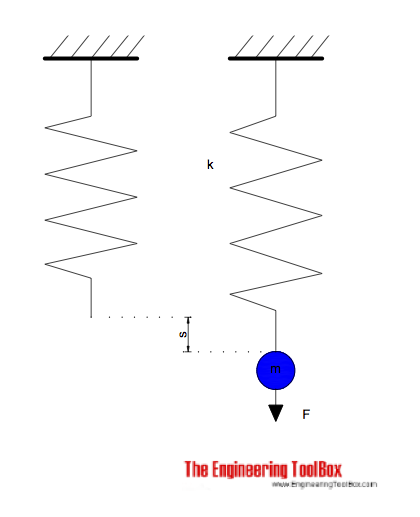Engineering ToolBox - Resources, Tools and Basic Information for Engineering and Design of Technical Applications!

# Hooke's Law

## Hooke's law - force, elongation and spring constant.The force required to extend or compress a spring can be expressed with Hooke's Law as

F = - k s                                    (1)

where

F = force (N)

k  = spring constant (N/m)

s = extension or compression distance (m)

#### Example - Car Suspension

The maximum compression of a car suspension with mass 2000 kg (500 kg on each wheel) shall not exceed 0.1 m.

The force acting on each spring can be calculated using Newton's Second Law

F = (500 kg) (9.81 m/s2)

= 4905 N

= 4.9 kN

The required spring constant for the suspension can be calculated with (1) as

k = - F / s

= - (4905 N) / (- 0.1 m)

= 49050 N/m

= 49 kN/m

Note that this is the value for the static load of the car. You may probably at least double the value for handling dynamic forces, potholes and similar.

### Hook's Law of Elasticity

In the generalized variation of Hooke's law it states that the strain/deformation of an elastic object or material is proportional to the stress applied to it. It may be expressed mathematically as

σ = E ε                              (2)

where

σ = stress (Pa)

E = Young's Modulus of Elasticity (Pa)

ε = strain (m/m)

## Related Topics

• Mechanics - Forces, acceleration, displacement, vectors, motion, momentum, energy of objects and more.
• Statics - Loads - forces and torque, beams and columns.

## Engineering ToolBox - SketchUp Extension - Online 3D modeling!

Add standard and customized parametric components - like flange beams, lumbers, piping, stairs and more - to your Sketchup model with the Engineering ToolBox - SketchUp Extension - enabled for use with the amazing, fun and free SketchUp Make and SketchUp Pro .Add the Engineering ToolBox extension to your SketchUp from the SketchUp Pro Sketchup Extension Warehouse!

Translate

## Privacy

We don't collect information from our users. Only emails and answers are saved in our archive. Cookies are only used in the browser to improve user experience.

Some of our calculators and applications let you save application data to your local computer. These applications will - due to browser restrictions - send data between your browser and our server. We don't save this data.

## Citation

• Engineering ToolBox, (2013). Hooke's Law. [online] Available at: https://www.engineeringtoolbox.com/hookes-law-force-spring-constant-d_1853.html [Accessed Day Mo. Year].

Modify access date.

. .

#### Scientific Online Calculator3 30